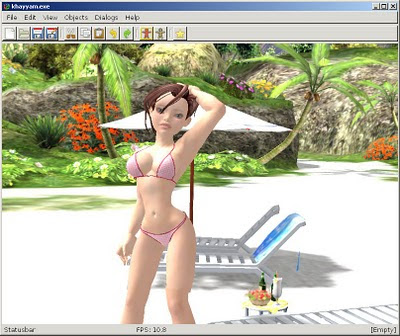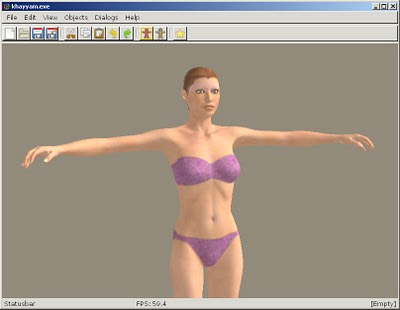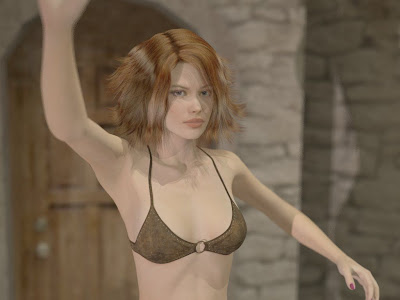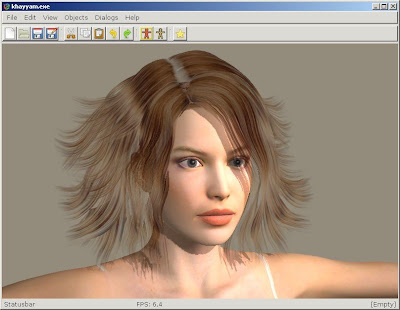## Sunday, February 27, 2011

### The Girl and full body morphs

Have spent last days by writing code for morph injection. While Khayyam has supported both standalone and ERC morphs for some time, they had until now to be specified in the main cr2 file.

ERC stands for "Enhanced Remote Control" and it is a feature of Poser/DAZ Studio to chain morphs (actually arbitrary channels) together, so changing the value of master dial propagates to different body parts by changing their channel values. Thus, for example, applying FBM (Full Body Morph) of The Girl, the master dial applies corresponding morph to head and at the same time moves eyes to slightly up and wider. Plus many-many things to other body parts.

The ERC feature is used extensively in DAZ version 4 figures (Victoria, Michael, Aiko, The Girl). Actually Aiko and The Girl do not have separate bodies at all anymore, but are defined as FBMs of Victoria. Most recent clothes have conforming morphs as well, but unlike the morphs of base figures they may be needed to be loaded manually from separate file. Thus the urgent need for Khayyam for applying extra morph files to objects (by poser slang it is called injection).

The result can be seen at the following screenshot:The Girl at the beach

Here you can see The Girl (Victoria 4 morph, so the body is actually the same as on previous Victoria pictures). Material is the original DAZ Studio "The Girl" texture set. Swimwear is Bucketload Sunny Bikini set, morphed to The Girl body. Background is beach scene from Sexy Beach 3.

Not everything works yet - for example The Girl FBM changes the sizes of some body parts like palms, and scaling is not implemented properly in Khayyam. To get the correct scaled hands, skeleton has to be modified (to move fingers along with scaled palm). Plus SmoothScale channel is not used, so the scaling boundaries are sharp.

## Wednesday, February 23, 2011

### New snapshot release 20110222

A new snapshot release 20110214 is available from Sourceforge page as both source tarball and precompiled Win32 binary.
• Fixed Poser deformation spheres so there are no "vertices going wild" anymore
• Materials can be imported as full sets from PZ2 files
• Poses can be applied to skeleton prom PZ2 files
• Transparency channel is applied automatically to Poser materials
• A new code for figure welding. As a result Chibibel is not broken anymore, but most body morphs do not work properly
And an obligatory screenshot - this time Chibibel was exercising in morning forest - but she is a bit worried by all you voyeurs.Chibibel in forest demonstrating imported Pz2 pose

Many things are still missing, but overall the program is taking shape nicely. So give it a try, if you have some unused models lying around and let me know about your mileage.

### Poses, poses, poses

Poser file formats are wonderful (or terrible) patchwork of figures and properties, endlessly extensible through a procedure called pose or morph injection. Basically you will take base figure (or extended one), add custom morphs, poses, materials and accessories (props), call it with fancy name and sell the extensions separately. As a result there is big and lively ecosystem of modelers and artists making living by selling virtual things for virtual worlds.

I am currently halfway through the implementation of those extension mechanisms for Khayyam. The most important ones - materials and poses mostly work now in CVS version and the results are really worth seeing.

Normally you start from plain base figure. As an example I use here the most famous one - DAZ Victoria 4 freely available from DAZ webpage.Victoria 4 base figure
Not very pretty - right?
Next let's load a predefined pose to make it a bit more lifelike.Victoria 4 posed

A bit better, but still ugly.
Now we import custom materials.Materials, swimwear and hair
Most texture sets are a bit ... realistic, so I added swimwear as well. Also imported hair. Imported objects are usually immediately skinnable - i.e. they follow the figure pose automatically, as you can see from swimwear.
There is more Khayyam can already do - like apply morphs.Closeup of face with eyes targeted and mouth morphed

Materials can be applied one on top of another - like eye color or makeup separately from main body texture. Transparent hair is a bit messed up in Khayyam, but will render properly if exported.
And last a quick render of the same figure with POV-Ray (different hair material and pose):Victoria 4 at beach - renderd with POV-Ray
That's all for today. Expect a release soon ;-)

## Friday, February 18, 2011

### Converting rotation matrix to arbitrarily ordered Euler angles

There is enhanced version of this algorithm available in new post.

The Matrix and Quaternion FAQ presents the straightforward method of converting rotation matrix to Euler angles. Still, it has two shortcomings.
• There is discontinuity close to Z rotation of 90 degrees, where X and Y rotations suddenly jump from close to zero to close to 180 degrees.
• It only shows the way to get angles in XYZ order (i.e. M = Mx * My * Mz).
Arbitrarily ordered angles are extremely useful for skeletal animation to give joints more natural deformations. But the main motivation was actually writing libmiletos container for Poser figures (cr2 file format). These have always bone rotations in twist/bend/bend order, while twist is defined as the rotation of the main axis of the bone. And as Poser figures have different bending algorithms for different rotations, the angles cannot be easily reordered.
So I have written slightly enhanced version for libelea.

Stable decomposition of matrix to Euler angles

First the original code.
It is derived from expanded form of rotation matrix:
```|  CE      -CF       D   0 |
M = |  BDE+AF  -BDF+AE  -BC  0 |
|  0        0        0   1 |

A,B are the cosine and sine of the X-axis rotation axis,
C,D are the cosine and sine of the Y-axis rotation axis,
E,F are the cosine and sine of the Z-axis rotation axis.
```
From it the following algorithm can be derived (using row-major matrix layout, so it has to be transposed for column-major OpenGL matrices):
```angleY = asin (m);
C =  cos (angleY);
if (fabs (C) > 0.005) {
trx    =  m / C;
try    = -m  / C;
angleX = atan2 (try, trx);
trx    =  m / C;
try    = -m / C;
angleZ = atan2 (try, trx);
} else {
angleX = 0;
trx    =  m;
try    =  m;
angleZ = atan2 (try, trx);
}
```
The problem is that when angleY goes from slightly below 90 degrees to slightly above 90 degrees, C changes sign and suddenly angles X and Y jump to different quadrant.

The enhanced version from libelea (also written for row-major order matrices):
```aY = asin (m);
aY2 = M_PI_F - aY;
if (fabs (m) != 1) {
C = cos (aY);
C2 = cos (aY2);
trX = m / C;
trY = -m / C;
aX = atan2 (trY, trX);
aX2 = atan2 (-m / C2, m / C2);
trX = m / C;
trY = -m / C;
aZ = atan2 (trY, trX);
aZ2 = atan2 (-m / C2, m / C2);
} else {
aX = aX2 = 0;
trX = m;
trY = m;
aZ = aZ2 = atan2 (trY, trX);
}
if ((aX * aX + aY * aY + aZ * aZ) < (aX2 * aX2 + aY2 * aY2 + aZ2 * aZ2)) {
angleX = aX;
angleY = aY;
angleZ = aZ;
} else {
angleX = aX2;
angleY = aY2;
angleZ = aZ2;
}
```
The only trick here is to calculate both Y angles satisfying the formula
```sin (aY) = c
```
and subsequently two versions of other angles too. Then the actual set of angles is chosen such, that the squared sum of all angles is lesser. This behaves extremely well as far as I have tested it.

Decomposition of matrix to arbitrarily ordered Euler angles

The code from libelea (matrices are again row-major order).
The order of axes is given as an array of 3 integers, whose values denote axes (i.e. {0,1,2} is XYZ, {1,0,2} is YXZ and so on.
```// We need either quaternion or axis/angle pair
// of given rotation
// Go for quaternion now
Quaternionf q = m.getRotationQuaternion ();
float sign =
((order == (order + 1)) ||
(order == (order + 1))) ? 1.0f : -1.0f;
// Array of identity axis vectors
static Vector3f iv[] = {
Vector3fX, Vector3fY, Vector3fZ
};
// Compose matrix that transforms to reordered axes space
Matrix3x4f s;
for (int row = 0; row < 3; row++) {
for (int col = 0; col < 3; col++) {
s[4 * row + col] = iv[order[row]][col];
}
}
for (int col = 0; col < 3; col++) {
s[8 + col] = sign * s[8 + col];
}
// Transform quaternion
// As we transform the first 3 values anyways,
// we can as well use vector transformation method here
s.transformVector3InPlace (q);
// Compose new rotation matrix from transformed quaternion
Matrix3x4f rs;
rs.setRotation (q);
// Decompose new matrix to Euler angles
rs.getEulerAngles (angles);
// We have to switch sign, if order was left-hand
angles *= sign;
```
The idea is to transform matrix in such way, that the reordered rotation axes of original matrix become XYZ rotation axes of transformed matrix and then perform trivial decomposition.

Have fun!

## Tuesday, February 15, 2011

### Valentine day release

A new snapshot release 20110214 is available from Sourceforge page as both source tarball and precompiled Win32 binary.
This time it is mostly about Poser/DAZ 3D file format support.
• Most joint properties work (there are slight inconsistencies with deformer spheres still)
• Body morphs kinda work, but the results may vary depending on model and morph
• Hair can be imported as poseable item (attached to head actor)
• Textures can include separate transparency maps
• Clothes can be attached as skins so they follow poses
Not everything is easily available from GUI yet, so property editor is must-be tool.DAZ Victoria 4 posed in Khayyam and rendered with POV-Ray
I have tested it mostly with Victoria 4 and Chibibel, so there can still be inconsistencies with other models. Victorial is the DAZ 3D base female model and freely available at moment. Chibibel used to be free also, but the promotion is over now.
Other than Poser file formats (cr2, pp2 and hr2), there are improvements in Collada file exporter, OBJ importer skeleton editor. Plus small bugfixes here and there.

## Sunday, February 13, 2011

### How to get quaternion from rotation matrix

For now probably the whole generation of programmers has learned the basics of projective geometry from the famous Matrix and Quaternion FAQ. I myself have consulted it numerous times while writing Khayyam (or more precisely Elea support library). But experimenting with the 3D transformations I found that while theoretically correct, the two most esoteric methods of the FAQ give suboptimal results. Namely - converting the rotation matrix to quaternion or Euler angles becomes unstable for certain rotations and - at least for Euler angles - does not give the most intuitive solution. So decided to write slightly enhanced variants.

The original code from FAQ:
T = 1 + mat + mat + mat
if ( T > 0.00000001 ) {
S = sqrt(T) * 2;
X = ( mat - mat ) / S;
Y = ( mat - mat ) / S;
Z = ( mat - mat ) / S;
W = 0.25 * S;
} if ( mat > mat && mat > mat ) {
S = sqrt( 1.0 + mat - mat - mat ) * 2;
X = 0.25 * S;
Y = (mat + mat ) / S;
Z = (mat + mat ) / S;
W = (mat - mat ) / S;
} else if ( mat > mat ) {
S = sqrt( 1.0 + mat - mat - mat ) * 2;
X = (mat + mat ) / S;
Y = 0.25 * S;
Z = (mat + mat ) / S;
W = (mat - mat ) / S;
} else {
S = sqrt( 1.0 + mat - mat - mat ) * 2;
X = (mat + mat ) / S;
Y = (mat + mat ) / S;
Z = 0.25 * S;
W = (mat - mat ) / S;
}
The problem lies in how the calculation method is switched, depending on the comparison of certain matrix elements. If, for two sequential rotations, the matrix values happen to be only slightly different, but fall into different calculation paths, the resulting quaternions will be very different and thus interpolating between them results in sudden twists. This should not happen, if your matrices are perfectly orthogonal - but if they come into existence as the result of many multiplications and (especially) inversions chances are good that they are not. Now add into the mix rotation angles close to 180 degrees, and the there will be distortions.

Stable variant from libelea (please notice, that while usually libelea has column-major order, packed 3x4 matrices, as used here, have row-major order. So to port the code to OpenGL matrices you have to transpose matrix):
/* Get normalized transformed unit vectors of rotated space */
Vector3f axx(Vector3f(m, m, m).normalize ());
Vector3f axy(Vector3f(m, m, m).normalize ());
Vector3f axz(Vector3f(m, m, m).normalize ());

/* Calculate transposition vectors */
/* I.e. how the unit vector endpoints will be transformed */
Vector3f tx = axx - Vector3fX;
Vector3f ty = axy - Vector3fY;
Vector3f tz = axz - Vector3fZ;

/* Find two biggest transpositions */
f32 txl2 = tx.length2 ();
f32 tyl2 = ty.length2 ();
f32 tzl2 = tz.length2 ();
Vector3f *a = &tx;
Vector3f *b = &ty;
if ((txl2 < tyl2) && (txl2 < tzl2)) {
a = &ty;
b = &tz;
} else if ((tyl2 < txl2) && (tyl2 < tzl2)) {
a = &tz;
b = &tx;
}

/* Get axis (cross product of two biggest transpositions)*/
Vector3f ax(*a * *b);
if (!ax.length2 ()) {
/* Zero rotation */
return Quaternionf0;
}
ax.normalizeSelf ();

/* Get vector perpendicular to axis and transform it */
Vector3f s(a->normalize ());
Vector3f t(m.transformVector3 (s));
t.normalizeSelf ();

/* Find rotation angle (between vector and its transform) */
Vector3f s_t(s * t);
f32 e = Vector3f::scalarProduct (s_t, ax);
if (e < 0) {
ax = -ax;
}
f32 theta = Vector3f::angle (s, t);
f32 sint2 = sinf (theta / 2);
f32 cost2 = cosf (theta / 2);
return Quaternionf(ax[X] * sint2, ax[Y] * sint2, ax[Z] * sint2, cost2);

The idea is, that any vector perpendicular to axis will be transformed to vector also perpendicular to axis. So we can get axis as the cross product between such vector and its transform.
Now, as you can see, this is much slower, involving the vector normalization, cross products and trigonometric functions. But hopefully you do not have to do this very often - I mostly only needed it for importing foreign animation formats and compacting rotation matrices for serializing.
I have used this method quite extensively and it has excellent stability.

Next time I'll show, how to avoid sudden instabilities if converting rotation matrix to Euler angles.

## Thursday, February 10, 2011

### DAZ Victoria 4

Have been trying to get DAZ 3D base model Victoria 4 to load and render in Khayyam. Things are slowly getting better, although it is not in very usable state yet.In-engine screenshot of Victoria 4 with Jamie hair

• Body is loaded normally and most joints behave well
• Morphs do not work yet
• Hair can be loaded only as static object (no morphing, not attached to skeleton)
• Textures can be applied (only diffuse, specular and transparency)
With some patience it can be made looking pretty good. Like most Poser models, the textures are either missing from base model, or so ugly they are pretty useless. So one has to apply textures one-by-one, using material overrides.POV-Ray rendering of Victoria 4 with Jamie hair

Getting Poser stuff to work is pretty high-priority now. Especially because Illusion does not seem to be planning to release anything noteworthy for some time. Meanwhile at places like Renderosity there is almost infinite amount of very high quality content available - although most of it is a bit too realistic for my taste.

## Monday, February 7, 2011

### Sara and Cyberdemon (and lighting)

I have been ill (sick if you are American) for the last week, so there was very little interesting things happening with Khayyam. But at last I am feeling better now, so hopefully more will come.

I took few evenings some weeks ago and fixed diffuse and specular lighting (and tangent map calculation). As as result, there is again a reason to read normal- and specular map textures for objects.Sara does not surrender easily (of course AK-47 itself is also legendary)

At least Doom creatures will look much better this way. Unfortunately Illusion games do not use normal maps, so there are no visible enhancements - unless you want to emulate the in-game plastic oily look.

But - it is possible to replace textures on Illusion (and other formats too) models with materialDNS node (look at tutorial for how exactly) - and this material node supports both normal and specular maps. So if someone wants to experiment, surface effects can be done with some effort.

There are still some big things to do for lighting - like cascading shadow maps, shadows for point and parallel lights and so on. Although immediately I want to play with skin shader instead...